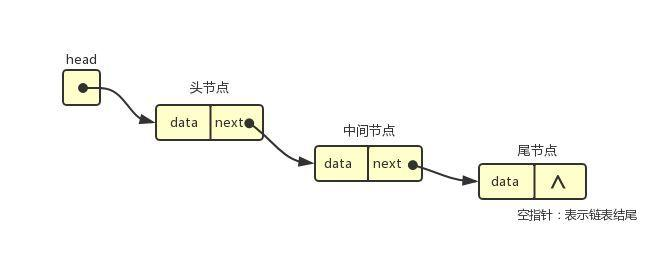• 编译期需要确定元素大小
• 数组在内存中是连续的，插入或者删除需要移动数组中其他数据

1、单向链表

``````#ifndef INT_LINKED_LIST

class Node {
public:
//构造函数，创建一个节点
Node(int el = 0, Node* ptr = nullptr) {
info = el;
next = ptr;
}
//节点的值
int info;
//下一个节点地址
Node* next;
};

class NodeList {
public:
//构造函数，创建一个链表，用于管理节点
NodeList() {
}
//节点插入到头部
//节点插入到尾部
//删除头部节点
//删除尾部节点
int  deleteFromTail();
//删除指定节点
void deleteNode(int);
private:
//头指针、尾指针
};

#endif
``````

• 节点插入到头部

• 节点插入到尾部

• 删除头部节点

• 删除尾部节点

• 删除指定节点

2、双向链表

``````#ifndef INT_LINKED_LIST

class Node {
public:
//构造函数，创建一个节点
Node(int el = 0, Node* p = nullptr, Node* q = nullptr) {
info = el;
pre  = p;
next = q;
}
//节点的值
int info;
//前一个节点地址
Node* pre;
//下一个节点地址
Node* next;
};

class NodeList {
public:
//构造函数，创建一个链表，用于管理节点
NodeList() {
}
//节点插入到头部
//节点插入到尾部
//删除头部节点
//删除尾部节点
int  deleteFromTail();
//删除指定节点
void deleteNode(int);
private:
//头指针、尾指针
};

#endif``````

• 删除尾部节点